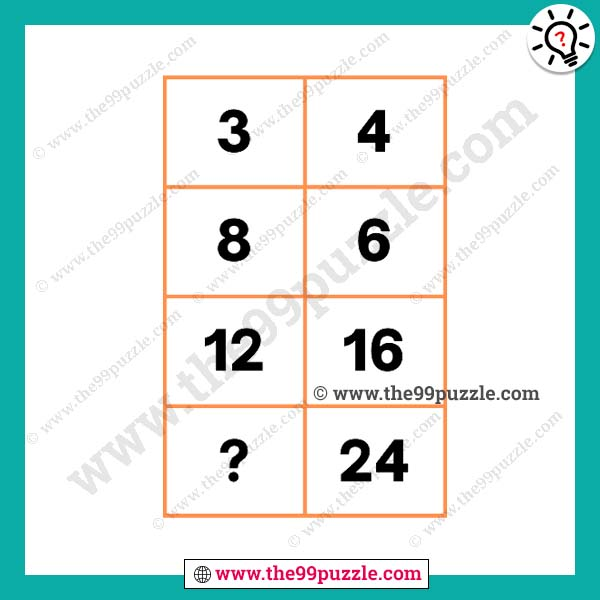# Mathematical missing number question with answer – Puzz268

This type of logical math problem is very challenging and interesting. Here in this picture, you see the box with some numbers. You have to solve the math logic and replace the question mark. Logical thinking and viral math equations can only solve the genius people. If you understand the logic you can easily find out the value of the mathematical missing number question.3×2=6

6×2=12

12×2=24

4×2=8

8×2=16

16×2=32